# Stats LECTURE 2.pptx

24 de Mar de 2023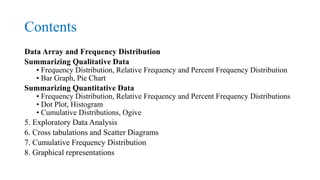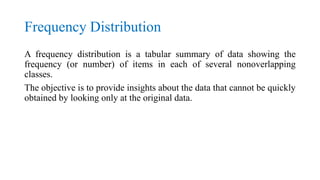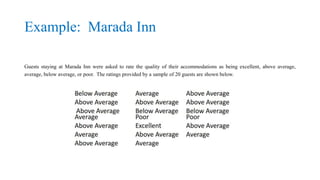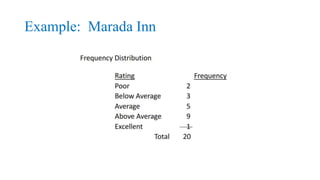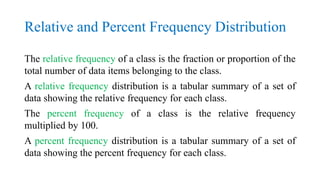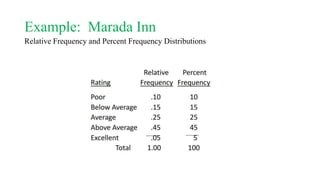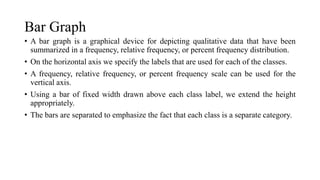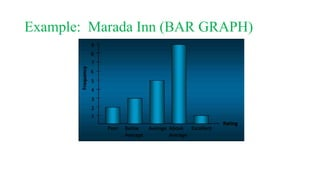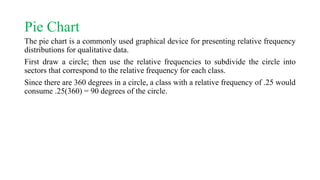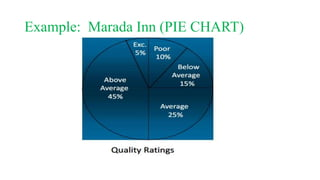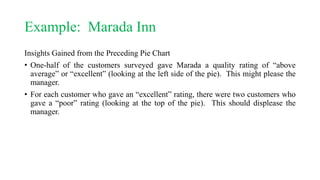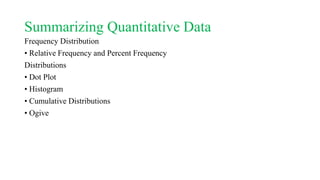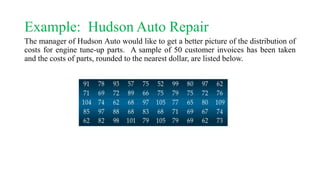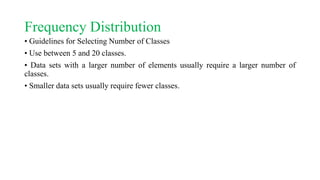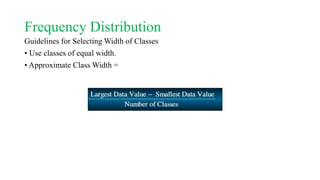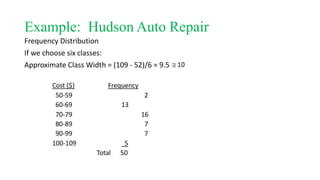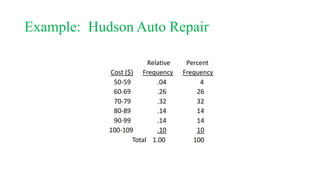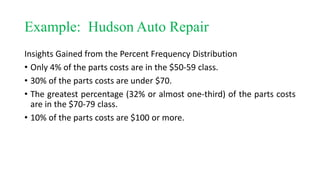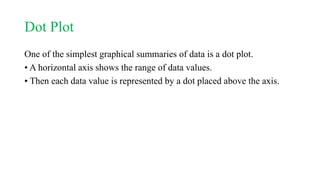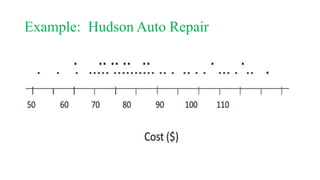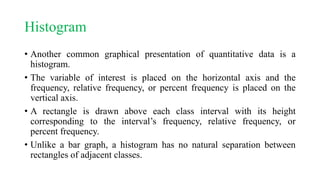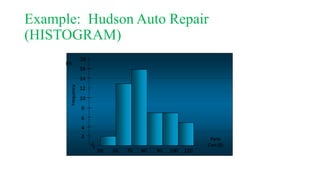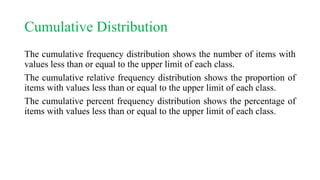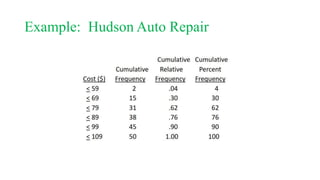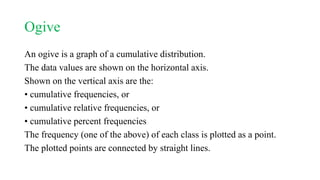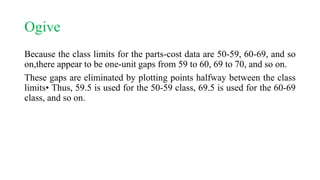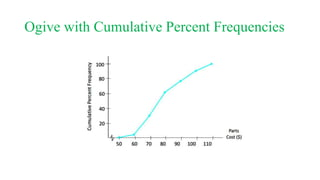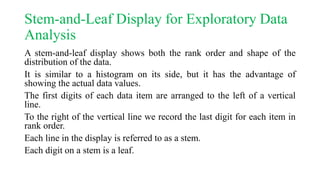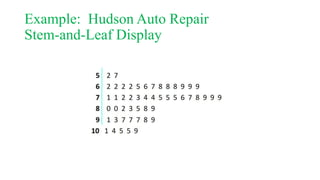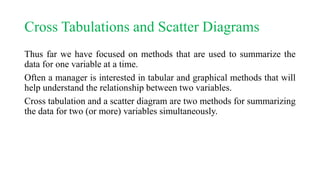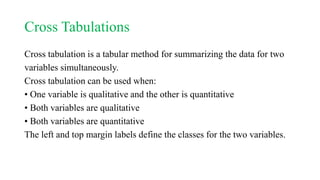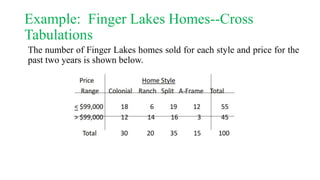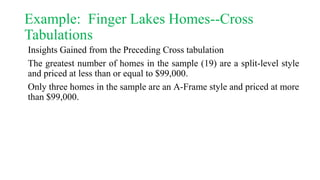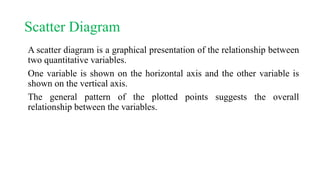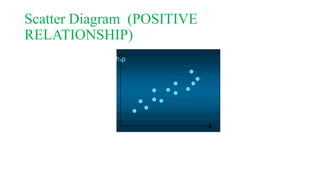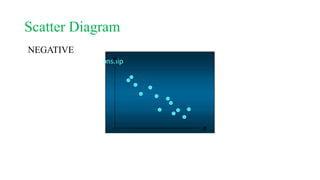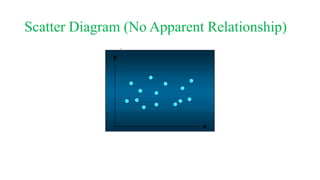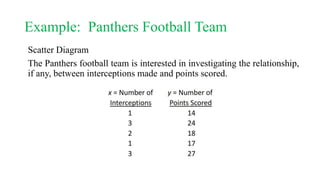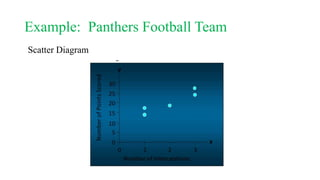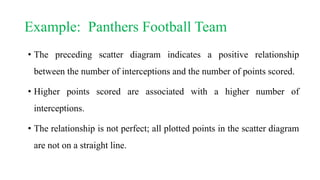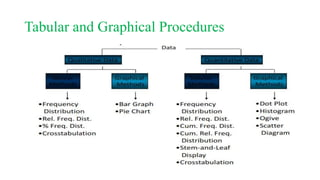1 de 42

### Stats LECTURE 2.pptx

1. Data Condensation and Presentation By Miss Kehkashan Nizam
2. Contents Data Array and Frequency Distribution Summarizing Qualitative Data • Frequency Distribution, Relative Frequency and Percent Frequency Distribution • Bar Graph, Pie Chart Summarizing Quantitative Data • Frequency Distribution, Relative Frequency and Percent Frequency Distributions • Dot Plot, Histogram • Cumulative Distributions, Ogive 5. Exploratory Data Analysis 6. Cross tabulations and Scatter Diagrams 7. Cumulative Frequency Distribution 8. Graphical representations
3. Frequency Distribution A frequency distribution is a tabular summary of data showing the frequency (or number) of items in each of several nonoverlapping classes. The objective is to provide insights about the data that cannot be quickly obtained by looking only at the original data.
4. Example: Marada Inn Guests staying at Marada Inn were asked to rate the quality of their accommodations as being excellent, above average, average, below average, or poor. The ratings provided by a sample of 20 guests are shown below.
6. Relative and Percent Frequency Distribution The relative frequency of a class is the fraction or proportion of the total number of data items belonging to the class. A relative frequency distribution is a tabular summary of a set of data showing the relative frequency for each class. The percent frequency of a class is the relative frequency multiplied by 100. A percent frequency distribution is a tabular summary of a set of data showing the percent frequency for each class.
7. Example: Marada Inn Relative Frequency and Percent Frequency Distributions
8. Bar Graph • A bar graph is a graphical device for depicting qualitative data that have been summarized in a frequency, relative frequency, or percent frequency distribution. • On the horizontal axis we specify the labels that are used for each of the classes. • A frequency, relative frequency, or percent frequency scale can be used for the vertical axis. • Using a bar of fixed width drawn above each class label, we extend the height appropriately. • The bars are separated to emphasize the fact that each class is a separate category.
9. Example: Marada Inn (BAR GRAPH)
10. Pie Chart The pie chart is a commonly used graphical device for presenting relative frequency distributions for qualitative data. First draw a circle; then use the relative frequencies to subdivide the circle into sectors that correspond to the relative frequency for each class. Since there are 360 degrees in a circle, a class with a relative frequency of .25 would consume .25(360) = 90 degrees of the circle.
11. Example: Marada Inn (PIE CHART)
12. Example: Marada Inn Insights Gained from the Preceding Pie Chart • One-half of the customers surveyed gave Marada a quality rating of “above average” or “excellent” (looking at the left side of the pie). This might please the manager. • For each customer who gave an “excellent” rating, there were two customers who gave a “poor” rating (looking at the top of the pie). This should displease the manager.
13. Summarizing Quantitative Data Frequency Distribution • Relative Frequency and Percent Frequency Distributions • Dot Plot • Histogram • Cumulative Distributions • Ogive
14. Example: Hudson Auto Repair The manager of Hudson Auto would like to get a better picture of the distribution of costs for engine tune-up parts. A sample of 50 customer invoices has been taken and the costs of parts, rounded to the nearest dollar, are listed below.
15. Frequency Distribution • Guidelines for Selecting Number of Classes • Use between 5 and 20 classes. • Data sets with a larger number of elements usually require a larger number of classes. • Smaller data sets usually require fewer classes.
16. Frequency Distribution Guidelines for Selecting Width of Classes • Use classes of equal width. • Approximate Class Width =
17. Example: Hudson Auto Repair Frequency Distribution If we choose six classes: Approximate Class Width = (109 - 52)/6 = 9.5
18. Example: Hudson Auto Repair
19. Example: Hudson Auto Repair Insights Gained from the Percent Frequency Distribution • Only 4% of the parts costs are in the \$50-59 class. • 30% of the parts costs are under \$70. • The greatest percentage (32% or almost one-third) of the parts costs are in the \$70-79 class. • 10% of the parts costs are \$100 or more.
20. Dot Plot One of the simplest graphical summaries of data is a dot plot. • A horizontal axis shows the range of data values. • Then each data value is represented by a dot placed above the axis.
21. Example: Hudson Auto Repair
22. Histogram • Another common graphical presentation of quantitative data is a histogram. • The variable of interest is placed on the horizontal axis and the frequency, relative frequency, or percent frequency is placed on the vertical axis. • A rectangle is drawn above each class interval with its height corresponding to the interval’s frequency, relative frequency, or percent frequency. • Unlike a bar graph, a histogram has no natural separation between rectangles of adjacent classes.
23. Example: Hudson Auto Repair (HISTOGRAM)
24. Cumulative Distribution The cumulative frequency distribution shows the number of items with values less than or equal to the upper limit of each class. The cumulative relative frequency distribution shows the proportion of items with values less than or equal to the upper limit of each class. The cumulative percent frequency distribution shows the percentage of items with values less than or equal to the upper limit of each class.
25. Example: Hudson Auto Repair
26. Ogive An ogive is a graph of a cumulative distribution. The data values are shown on the horizontal axis. Shown on the vertical axis are the: • cumulative frequencies, or • cumulative relative frequencies, or • cumulative percent frequencies The frequency (one of the above) of each class is plotted as a point. The plotted points are connected by straight lines.
27. Ogive Because the class limits for the parts-cost data are 50-59, 60-69, and so on,there appear to be one-unit gaps from 59 to 60, 69 to 70, and so on. These gaps are eliminated by plotting points halfway between the class limits• Thus, 59.5 is used for the 50-59 class, 69.5 is used for the 60-69 class, and so on.
28. Ogive with Cumulative Percent Frequencies
29. Stem-and-Leaf Display for Exploratory Data Analysis A stem-and-leaf display shows both the rank order and shape of the distribution of the data. It is similar to a histogram on its side, but it has the advantage of showing the actual data values. The first digits of each data item are arranged to the left of a vertical line. To the right of the vertical line we record the last digit for each item in rank order. Each line in the display is referred to as a stem. Each digit on a stem is a leaf.
30. Example: Hudson Auto Repair Stem-and-Leaf Display
31. Cross Tabulations and Scatter Diagrams Thus far we have focused on methods that are used to summarize the data for one variable at a time. Often a manager is interested in tabular and graphical methods that will help understand the relationship between two variables. Cross tabulation and a scatter diagram are two methods for summarizing the data for two (or more) variables simultaneously.
32. Cross Tabulations Cross tabulation is a tabular method for summarizing the data for two variables simultaneously. Cross tabulation can be used when: • One variable is qualitative and the other is quantitative • Both variables are qualitative • Both variables are quantitative The left and top margin labels define the classes for the two variables.
33. Example: Finger Lakes Homes--Cross Tabulations The number of Finger Lakes homes sold for each style and price for the past two years is shown below.
34. Example: Finger Lakes Homes--Cross Tabulations Insights Gained from the Preceding Cross tabulation The greatest number of homes in the sample (19) are a split-level style and priced at less than or equal to \$99,000. Only three homes in the sample are an A-Frame style and priced at more than \$99,000.
35. Scatter Diagram A scatter diagram is a graphical presentation of the relationship between two quantitative variables. One variable is shown on the horizontal axis and the other variable is shown on the vertical axis. The general pattern of the plotted points suggests the overall relationship between the variables.
36. Scatter Diagram (POSITIVE RELATIONSHIP)
37. Scatter Diagram NEGATIVE
38. Scatter Diagram (No Apparent Relationship)
39. Example: Panthers Football Team Scatter Diagram The Panthers football team is interested in investigating the relationship, if any, between interceptions made and points scored.
40. Example: Panthers Football Team Scatter Diagram
41. Example: Panthers Football Team • The preceding scatter diagram indicates a positive relationship between the number of interceptions and the number of points scored. • Higher points scored are associated with a higher number of interceptions. • The relationship is not perfect; all plotted points in the scatter diagram are not on a straight line.
42. Tabular and Graphical Procedures